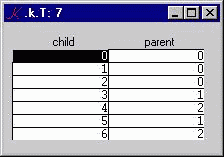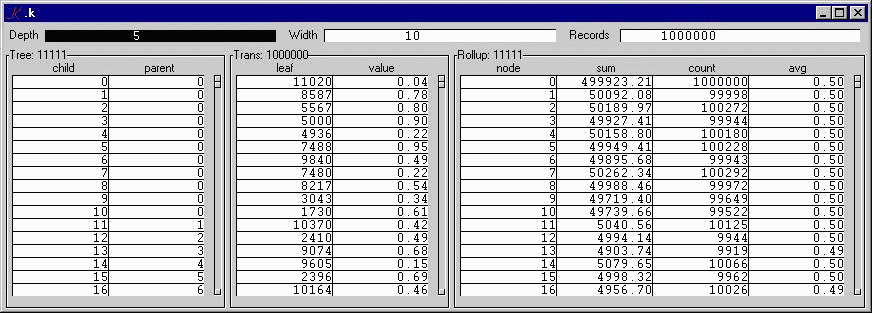# Hierarchical Rollup

## Problem

Given a set of transactions and a method for assigning each transaction to the leaf of a tree, produce the sum, count, and average of transaction values at each node of the tree.

## Representation

We can represent a tree as a table of child-parent records. For example, the tree T:

```	0
1
3
5
2
4
6
```

can be represented as the table:We need two numbers to generate balanced trees like T: Depth, the number of nodes from the root to any leaf, and Width, the number of branches at each node.

We can represent the transactions as a table U:U contains ten transaction records. The leaf column maps the value to a leaf in T. In this example, there are six transactions assigned to leaf 3, one to leaf 4, two leaf 6, and one to leaf 5.

We need one number to generate transaction tables like U: Records, the number of transaction records.

The rollup table R has four columns: node, sum, count, and avg. Each record in R maps calculated values to a node in T. For our example above, R looks like this:## Solution

The code is very straightforward:

```	Records:1000000
Width:10
Depth:5

Tree..d:".((`child;c);(`parent;,/0,{(#x)#y}':(0,+\\_ Width^!Depth-1)_ c:!+/_ Width^!Depth))"
Trans..d:".((`leaf;c Records _draw#c:Tree[`child]_dvl?Tree`parent);(`value;Records _draw 0))"

Rollup..d:"
p:Tree[`parent]\\'!#Tree`parent
g:Tree[`child]?/:Trans`leaf
f:{@[x;p;y;@[x;g;y;z]]}
s:f[(#p)#0.;+;Trans`value]
c:f[(#p)#0;+;1]
.((`node;Tree`child);(`sum;s);(`count;c);(`avg;s%c))"

.k..c:`form
.k..a:(`Depth`Width`Records;`Tree`Trans`Rollup)
`show\$`.k```

Depth, Width, and Records are input fields. If we type a new value into Depth or Width, Tree recalculates itself. If we type a new value into Records, Trans recalculates itself. If Tree or Trans changes, Rollup recalculates itself.

Tree, Trans, and Rollup are defined as K dependencies: whenever any variable used in the definition changes, the definiens is invalidated; when a variable is on the screen, if it becomes invalid, it is immediately validated by evaluating its definition.

Tree depends on Width and Depth. Trans depends on Records. Rollup depends on Trans and Tree.

The last three lines specify the GUI attributes class and arrangement, and show the set of variables.